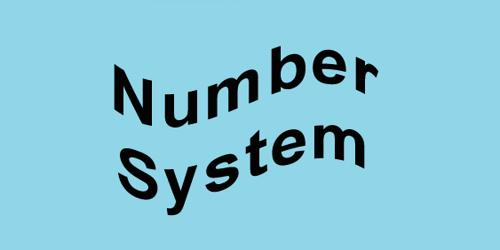Mathematics

# Number SystemFrom the beginning of civilization idea of counting developed in the human being. From then onward different counting systems have developed according to the need and convenience. In arithmetical processes, digital system is a process which uses different units independently, for example, hands, fingers, digit (0, 1, 3, …) etc. A full number can be expressed by using this unit or units independently in a bundle.

The process of writing a number is called number system. A number system is a collection of various symbols which are called digits. Different symbols to prepare number are arithmetic. By the help of this system, any quantity can be expressed, for example in decimal system 507 number is composed of three separate figures as 5, 0, 7. In number system, some fixed figures or numbers can be arranged following some rules and different numbers can be found. Using different rules like summation, subtraction, multiplication, division etc. different counting can be performed by these numbers or figures.

Different types of Number System –

1. Binary: The numbers that are made in Binary Number System are made up of 0 or 1.
2. Decimal: The number system that we follow is called as Decimal Number System where the base is 10.